# Neural Networks and Deep Learning（五）为什么深度神经网络难以训练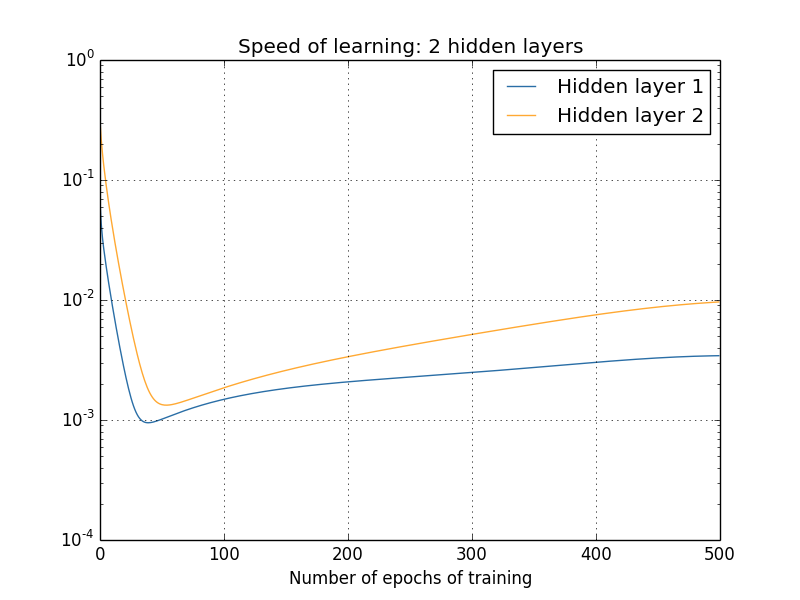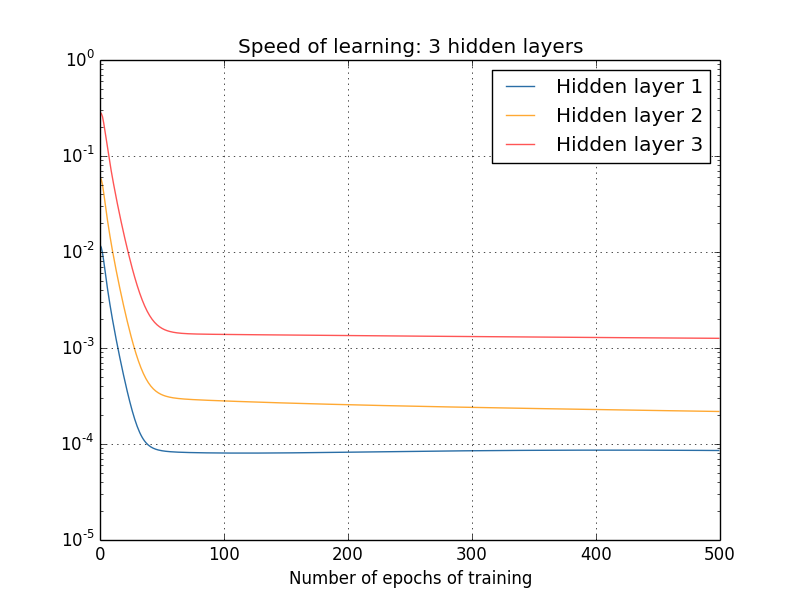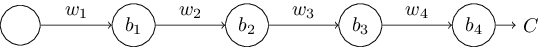$\begin{eqnarray}\frac{\partial C}{\partial b_1} = \sigma'(z_1) \, w_2 \sigma'(z_2) \,w_3 \sigma'(z_3) \, w_4 \sigma'(z_4) \, \frac{\partial C}{\partial a_4}.\tag{1}\end{eqnarray}$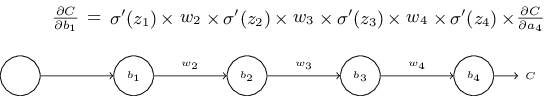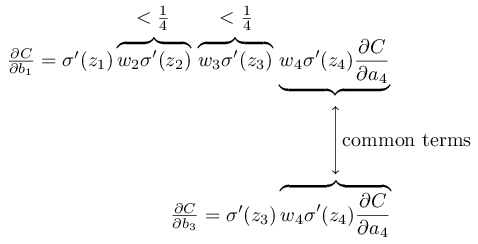This site uses Akismet to reduce spam. Learn how your comment data is processed.